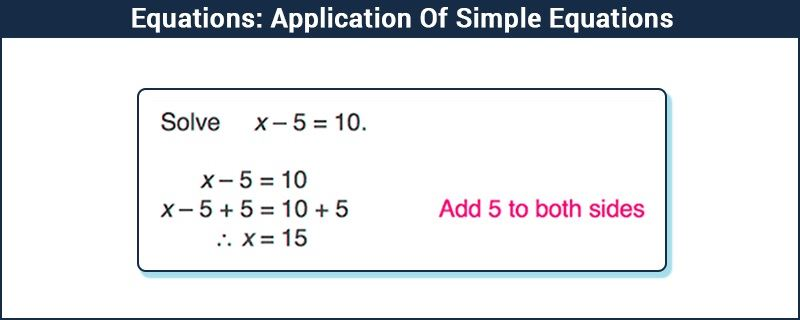# Simple Equations and Its Applications

In our day to day lives, we generally come across many situations where we observe simple equations and its applications.

## What is Simple Equation?

A mathematical equation which represents the relationship of two expressions on either side of the sign. It mostly has one variable and equal to symbol. Example: 2x – 4 = 2. In the given example, x is a variable.

Before understanding this, let us see an example, to visualize and interpret the meaning of simple equations.Suppose, we have an equation, 12x + 40 = 100. What is the solution to this equation and what is the meaning? As we have already learned through the given image, how to solve simple equations, we would not go in details of solving. The solution of the above linear equation,(12x +40 =100) is x=5.

## Applications of Simple Equations

But now, the question is, how to interpret the meaning of the above equation. Let’s learn the applications of Simple Equation through an example.

Let us assume a situation, in which you go to the market to buy mangoes in a supermarket. Your mother gave you Rs.100 and said that you can keep Rs.40 for buying chocolates and for the remaining amount, you need to buy mangoes. When you went to the market, you found out that the price of one kg of mangoes was Rs.12. So, the final question is how much mangoes you should buy, so that everyone is satisfied.

Recall the concept of simple equations from previous classes and proceed. Let us assume that you have to purchased kg of mangoes, so the total amount of money that you will be spent on buying mangoes will be Rs.12x. Now, you have Rs.40 for yourself, so you have an equation:

12 x + 40 = 100
12x = 100 – 40
12x = 60
x = 60/12 = 5

This is the same equation as above. Now when you will solve this, you will get to know the value of 5kg of mangoes. It means that you can buy 5 kg mangoes and spend Rs.40 on buying chocolates.
This is one of the general applications of simple equations. We can consider any other situation, where any unknown is involved and we will surely find the application of simple equations in that particular situation.

### Transposition

The process of moving a term from one side of the equation to the other side is called transposing. It is the same as adding and subtracting a number from both sides of the equation.

Ex. Solve 5x + 9 = 19

-> Transposing 9 to the other side we get,

-> 5x = 19 – 9

-> 5x = 10

Transposing 5 to the other side we get,

x = 10/5

x = 2

Hence, it could be stated without doubt that the use of simple equations are applicable everywhere and not just restricted to classrooms.

## Video Lesson